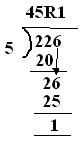Name: ___________________Date:___________________

 Email us to get an instant 20% discount on highly effective K-12 Math & English kwizNET Programs!

### Grade 3 - Mathematics10.11 Division Review Test

 Q 1: Work out the following and choose the correct answer.12R214R614R2 Q 2: 30 divided by 3=3151210 Q 3: In the division problem shown the answer is ____.Answer: Q 4: 670/10Answer: Q 5: In the division problem the remainder is ____.Answer: Q 6: 27 divided by 3=1265329 Q 7: 14 divided by 2=7684 Q 8: Work out the following and choose the correct answer.33R433R723R4 Question 9: This question is available to subscribers only! Question 10: This question is available to subscribers only!

#### Subscription to kwizNET Learning System costs less than \$1 per month & offers the following benefits:

• Unrestricted access to grade appropriate lessons, quizzes, & printable worksheets
• Instant scoring of online quizzes
• Progress tracking and award certificates to keep your student motivated
• Unlimited practice with auto-generated 'WIZ MATH' quizzes